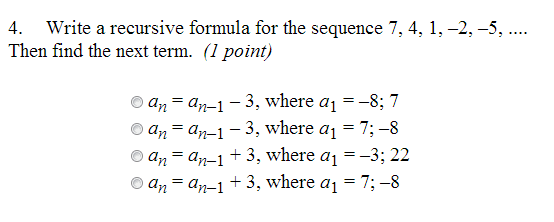1
0
watching
544
views10 Nov 2019

#Write a recursive formula for the sequence 7, 4, 1, -2, -5,.... Then find the next term. a n = a n-1 - 3, where a1 = -8; 7 a n = a n-1 - 3, where a1 = 7; -8 a n = a n-1 + 3. where a1 = -3; 22 a n = a n-1 + 3. where a1 = 7; -8